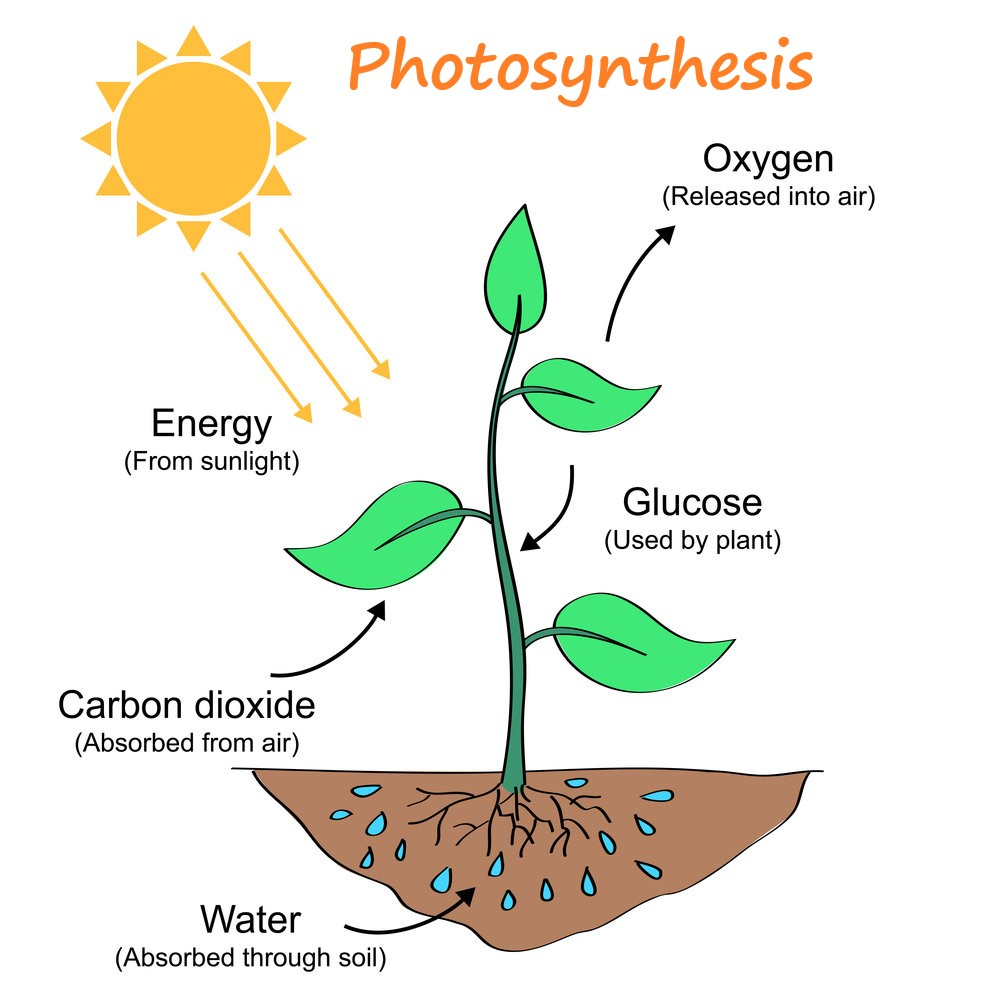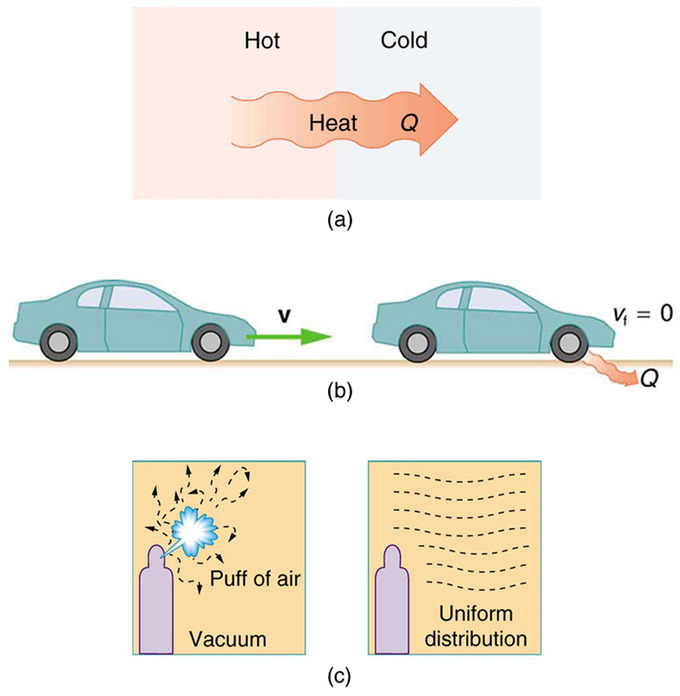# CBSE Class 11 Physics Chapter 12 Revision Notes

Chapter 12: Thermodynamics Revision Notes

• Thermodynamics is the branch of physics that studies the transformation of heat into other forms of energy and vice versa.Source

• The science of thermodynamics is a macroscopic one. It focuses on bulk systems rather than the molecular structure of matter.
• A thermodynamic system is a collection of an extremely large number of atoms or molecules confined within certain boundaries and having specific pressure (P), volume (V), and temperature (T) values.

Thermal Equilibrium

A thermodynamic system is in equilibrium if the macroscopic variables that characterize it, such as pressure, volume, temperature, mass composition, and so on, do not change over time. The temperatures of the two systems are equal in thermal equilibrium.

• Zeroth Law of Thermodynamics

This law establishes thermal equilibrium and makes temperature a tool for determining of equilibrium. “If two systems are in thermal equilibrium with a third system, then those two systems are in thermal equilibrium with each other,” says this law.

• Heat, Work, and Internal Energy

Heat is the energy that is transferred between a system and its surroundings when there is a temperature difference between the two.

— When a body or a system moves a certain distance in the direction of an applied force, it is said to be doing work. It’s written as dW = PdV, where P is the cylinder’s gas pressure.

— If we consider a large number of molecules in a bulk system, the internal energy of the system is equal to the sum of the kinetic and potential energies of these molecules.

• First Law ofThermodynamics

The first law of thermodynamics is the universal law of energy conservation that applies to all systems. “The total heat energy change in any system is the sum of the internal energy change and the work done,” says this law.

When a given amount of heat, dQ, is applied to a system, a portion of it is used to increase internal energy, dU, and a portion is used to perform external work, dW, resulting in dQ = dU + dW.

• Variables of Thermodynamic State

A system’s thermodynamic state variables are the parameters that describe the system’s equilibrium states. The values of pressure, volume, temperature, mass, and composition, for example, completely define the equilibrium state of a gas.

• Equation of State: The state equation depicts the relationship between a system’s state variables. For example, the equation of state for an ideal/perfect gas is PV = RT, where g denotes the number of moles of gas and R denotes the gas constant for one mole of gas.
• There are two types of thermodynamic state variables: extensive and intensive.
• Intensive variables do not indicate the size of the system, whereas extensive variables do.
• Pressure, temperature, and density are intensive variables, while volume, mass, and internal energy of a system are extensive variables.

## Processes of Thermodynamics

• Thermodynamic process refers to any process in which the thermodynamic variables of a thermodynamic system change.
• Quasi-static processes are those that are sufficiently slow and do not involve accelerated piston motion or a large temperature gradient.
• The change in pressure, volume, or temperature of the system during this process is extremely small.
• Thermodynamic Process: An isothermal change is defined as a change in pressure and volume of a gas without a change in temperature. There is a free exchange of heat between the gas and its surroundings during such a change.
• Adiabatic Process is a term used to describe a process in which there is no exchange of heat energy between the gas and its surroundings.

## Process that can be reversed

• A reversible process is one that can retrace its steps so that the system passes through the same states again; otherwise, it is irreversible.
• Irreversibility is caused by two factors:

(i) Non-equilibrium states are created by a variety of processes, such as free expansion or an explosive chemical reaction.

(ii) Friction, viscosity, and other dissipative effects are present in almost all processes.Source

• Second Law of Thermodynamics

The second law of thermodynamics is a principle that forbids certain phenomena that are consistent with the first law of thermodynamics.

The two statements of the second law of thermodynamics are as follows.

Kelvin-Planck Statement:

It is impossible to build a cycle engine that extracts heat from a hot body and converts it completely into work without any change, i.e., a 100 percent conversion of heat into work is impossible.

Clausius’s Proposition:

• It is impossible for a self-acting machine to transfer heat from a cold body to a hot body without the assistance of external energy.
• In other words, heat cannot flow from a colder to a hotter body on its own.
• A heat engine is a device that causes a system to go through a cyclic process that converts heat to work.
• A heat engine is made up of three parts: a heat source, a heat sink, and a working substance.
]]>